The LP Procedure
 RANGE Statement

RANGE variable ;

For the dense input format, the RANGE statement identifies the variable in the problem data set that contains the range coefficients. These coefficients enable you to specify the feasible range of a row. For example, if theth row is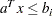and the range coefficient for this row is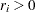, then all values ofthat satisfy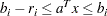are feasible for this row. Table 5.3 shows the bounds on a row as a function of the row type and the sign on a nonmissing range coefficient.

 Bounds_TYPE_ Lower Upper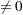LE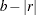GE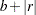EQ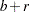EQIf you include a range variable in the model and have a missing value or zero for it in a constraint row, then that constraint is treated as if no range variable had been included.

If the RANGE statement is omitted, the LP procedure assumes that the variable named _RANGE_ contains the range coefficients.

For the sparse input format, the RANGE statement gives the name of a column in the problem data set that contains the range constants. If the RANGE statement is omitted, then the LP procedure assumes that the column named _RANGE_ or the column with the 'RANGE' keyword in the problem data set contains the range constants.Previous Page | Next Page | Top of Page